# NCERT Solutions for Class 10 Maths Chapter 8 Introduction to Trigonometry

NCERT Solutions for Class 10 Maths Chapter 8 Introduction to Trigonometry

Students can benefit from NCERT Solutions for Class 10 Maths Chapter 8 Introduction to Trigonometry because it will help them understand the concepts and perform well in the CBSE Class 10 2023 examination. The NCERT Solutions are created and reviewed by subject experts to ensure that they cover all of the questions in the textbook.

These NCERT Solutions are based on the most recent revisions to the CBSE Syllabus for 2021-22 and its accompanying guidelines and the 2023 exam pattern. The NCERT Solutions for Class 10 Maths provide a solid foundation for all concepts covered in each chapter. Students can get answers to their questions and learn the fundamentals in this chapter. Students can also use these NCERT Solutions for Class 10 Maths Chapter 8 to solve the difficult problems in each exercise.

NCERT Solutions for Class 10 Maths Chapter 8 Introduction to Trigonometry | Free PDF Download

Out of 80 marks (combined) for Class 10 CBSE Maths, 12 marks are assigned to Chapter 8, "Trigonometry." The paper is divided into four sections. Each section has a different set of marks, with 1 mark, 2 marks, 3 marks, and 4 marks assigned to the questions. This chapter will most likely include at least 2-3 mandatory questions. The main topics covered in this chapter are as follows: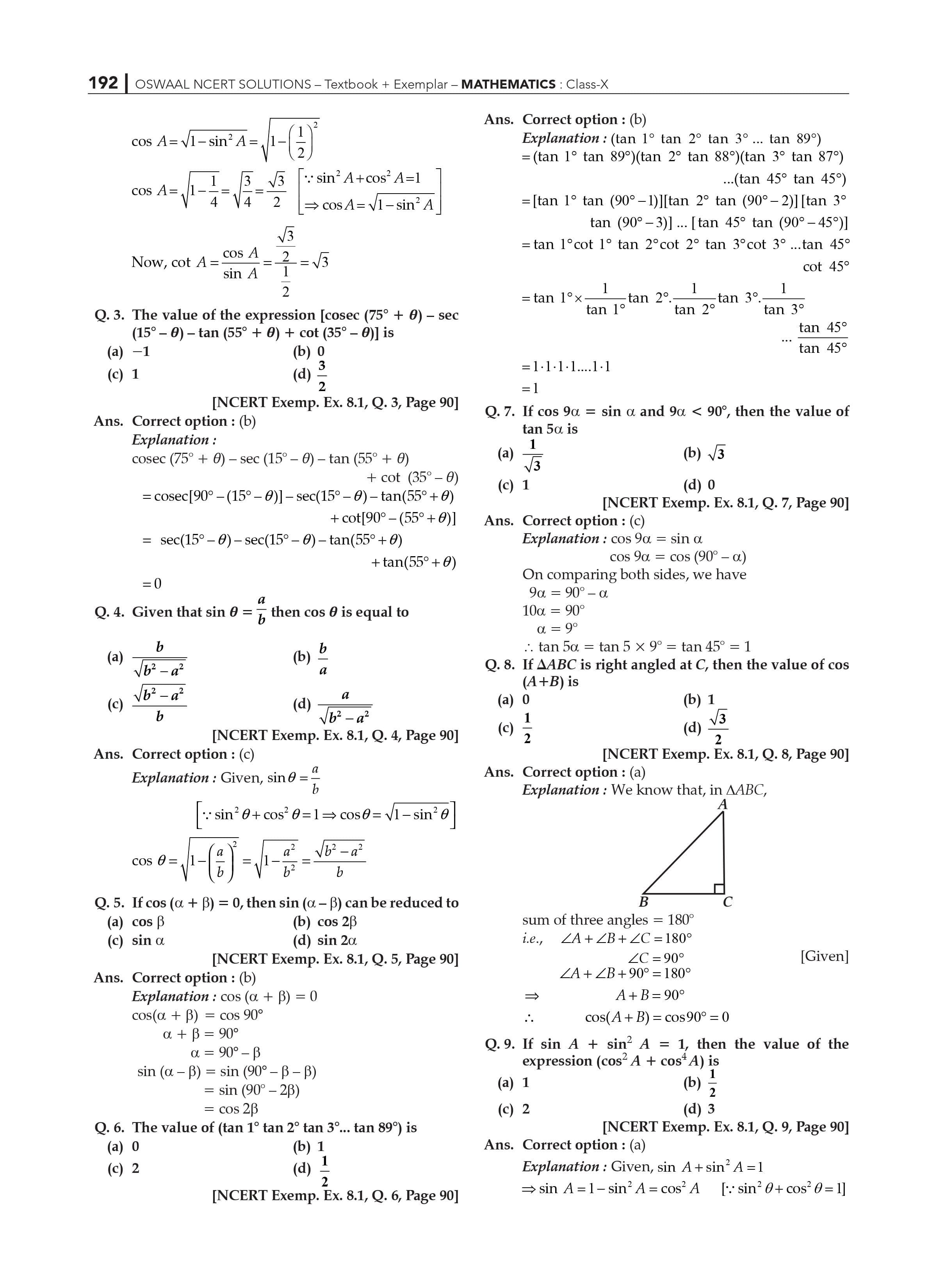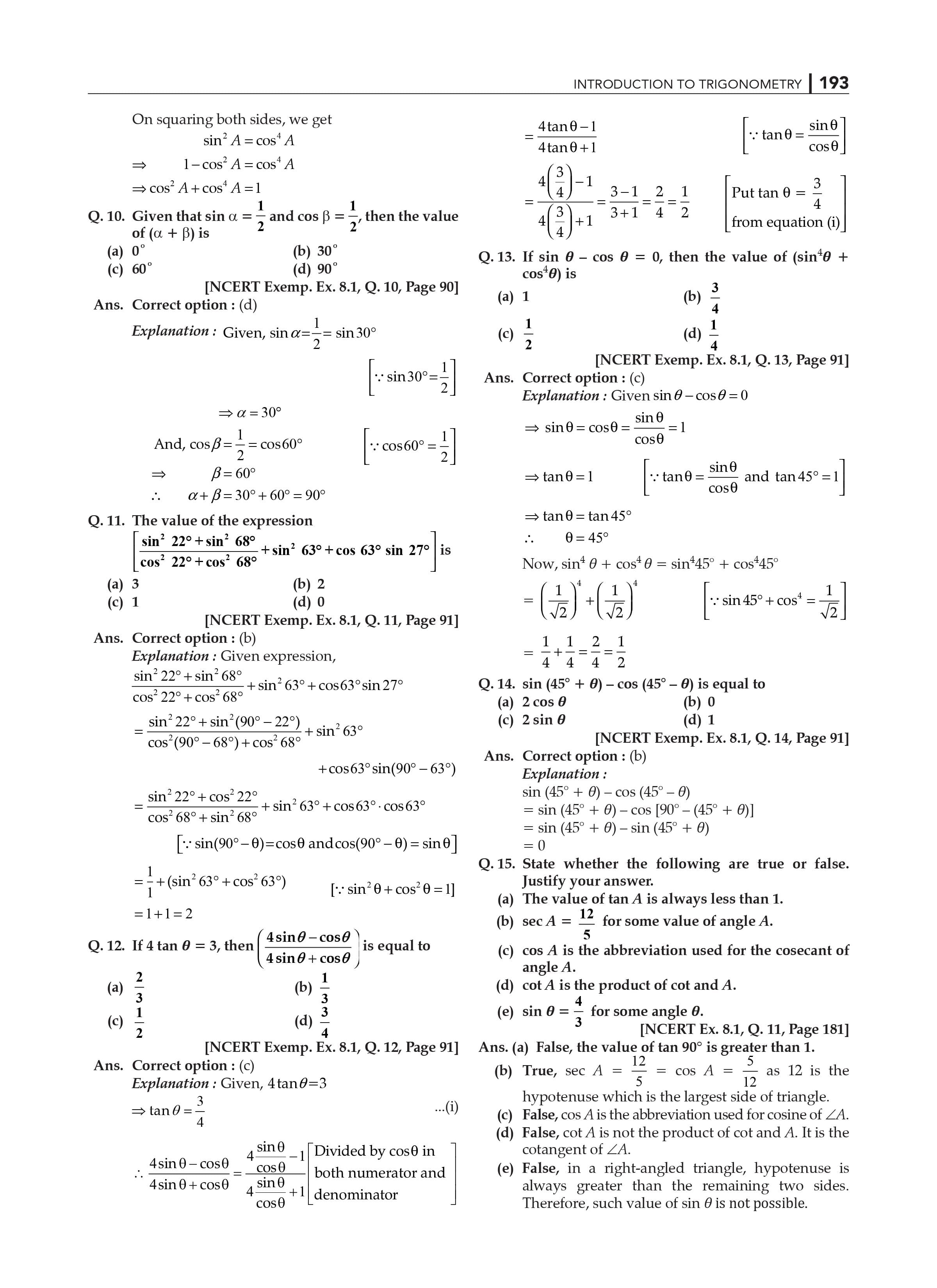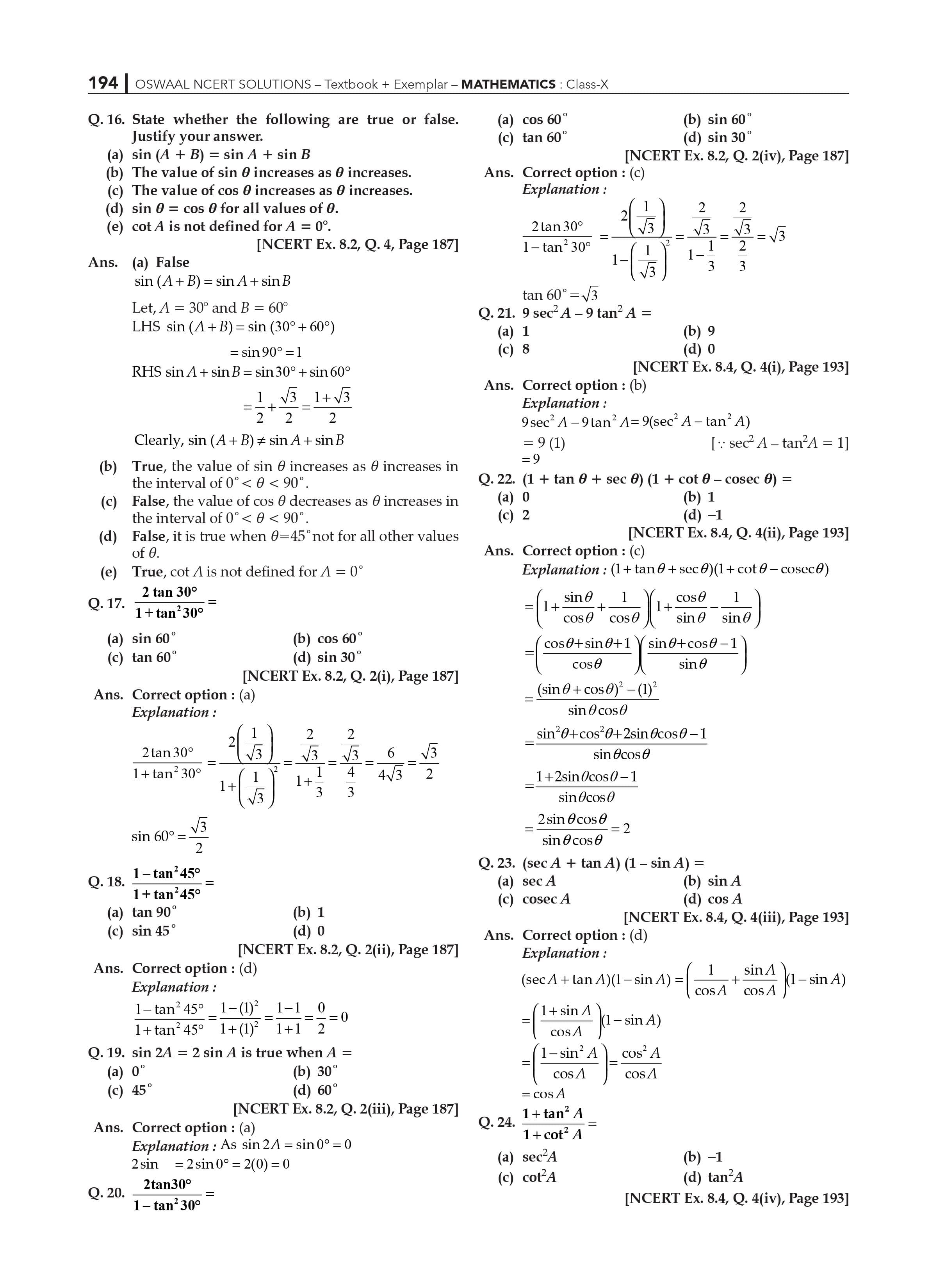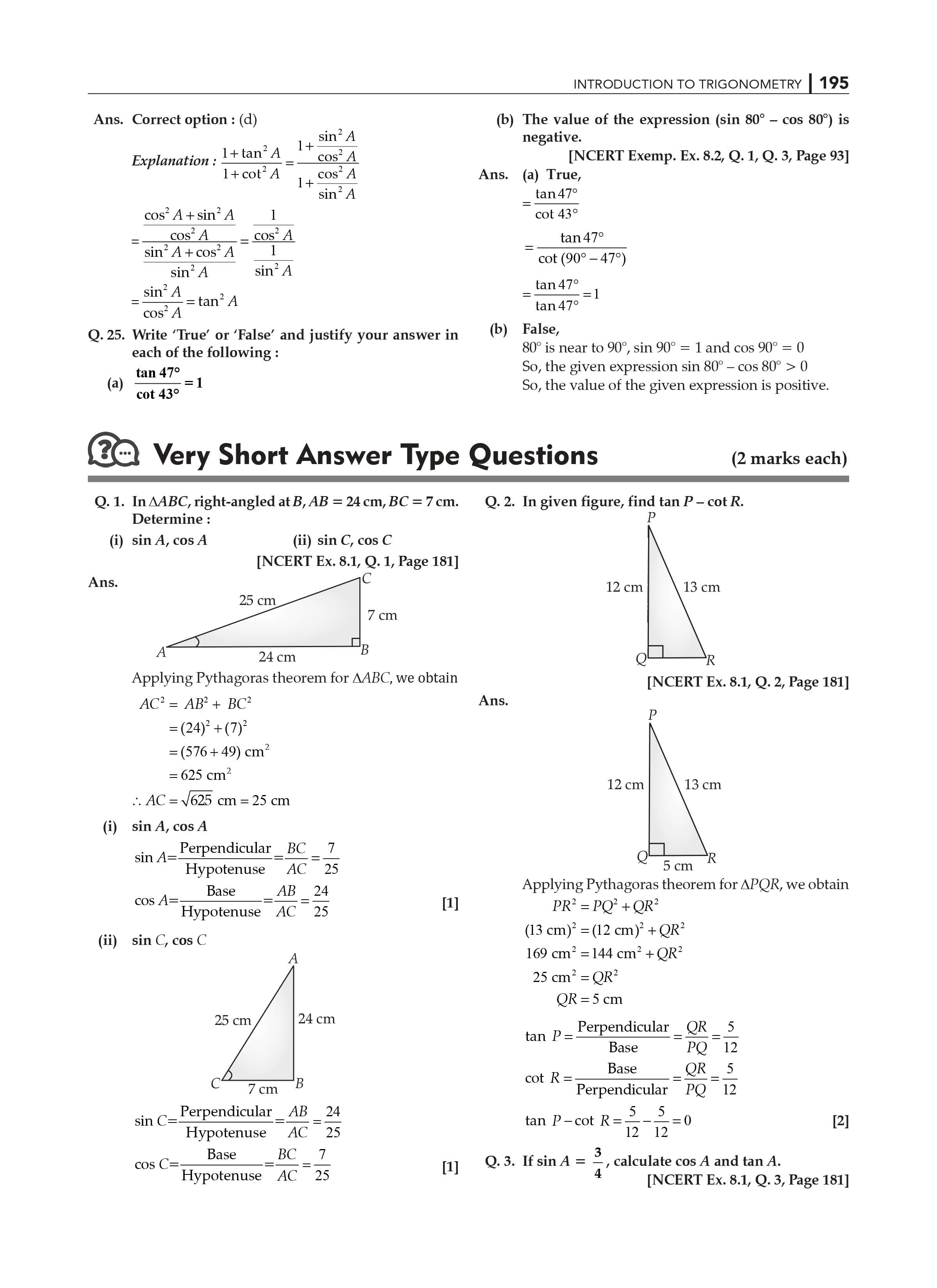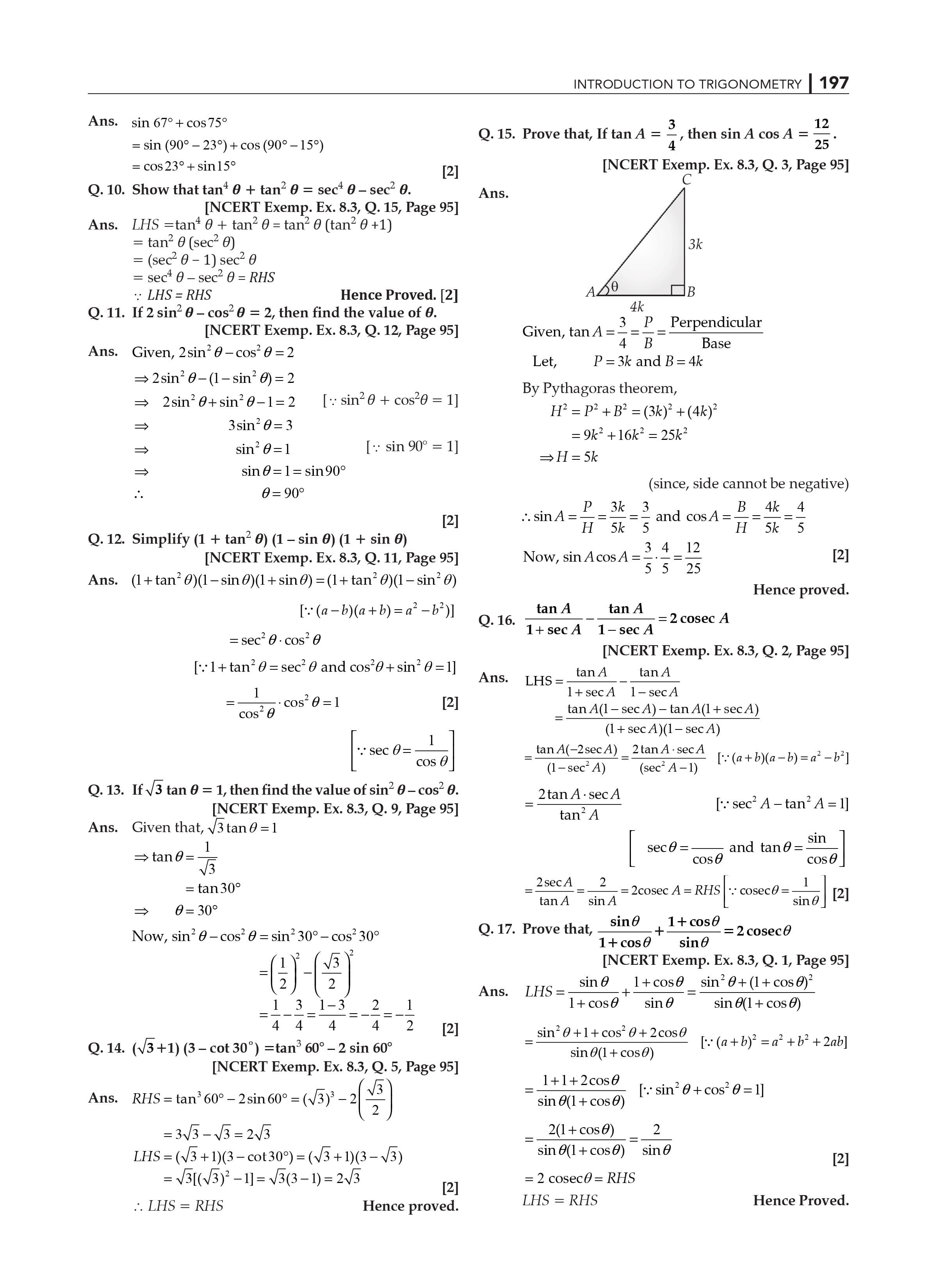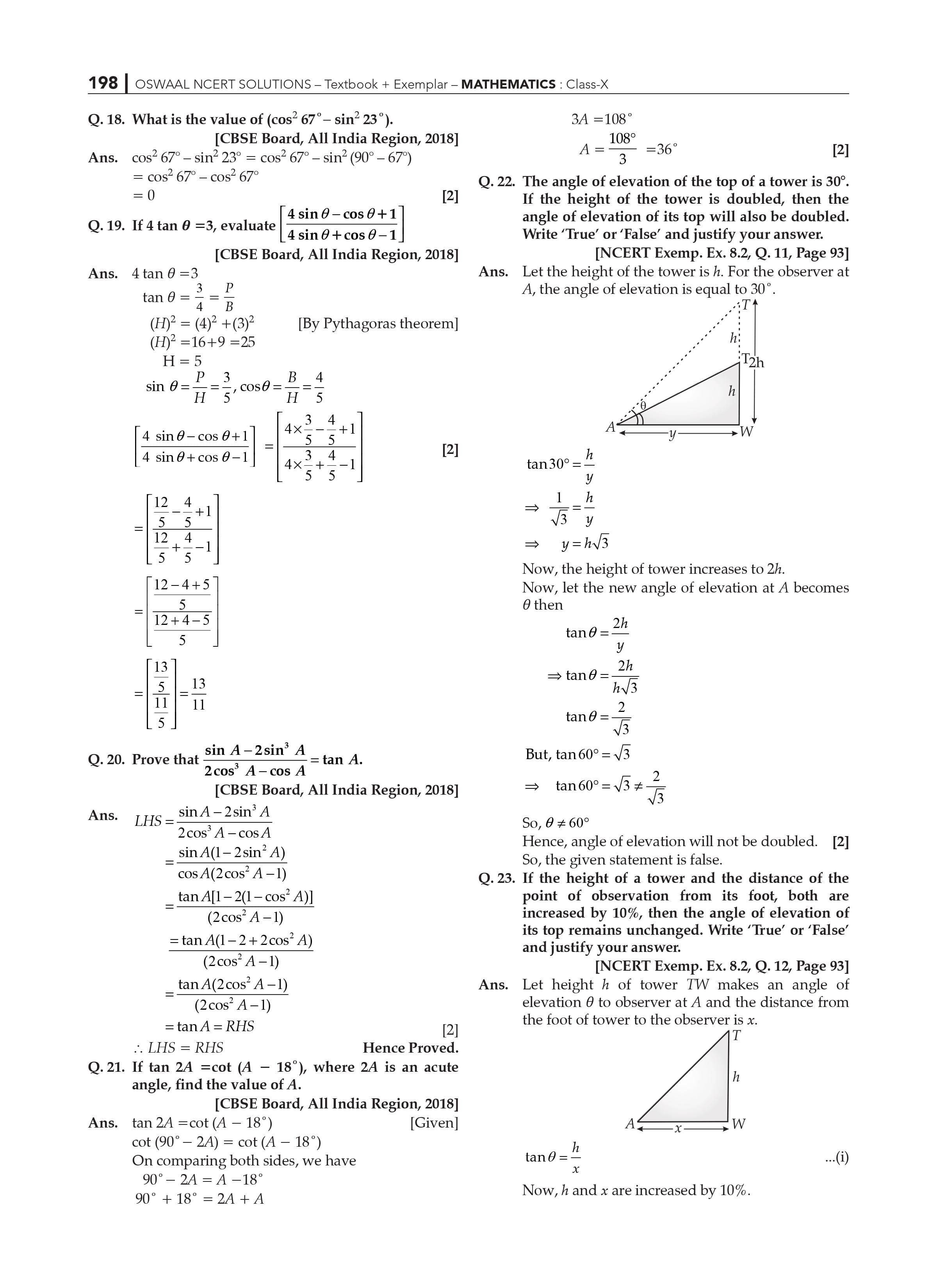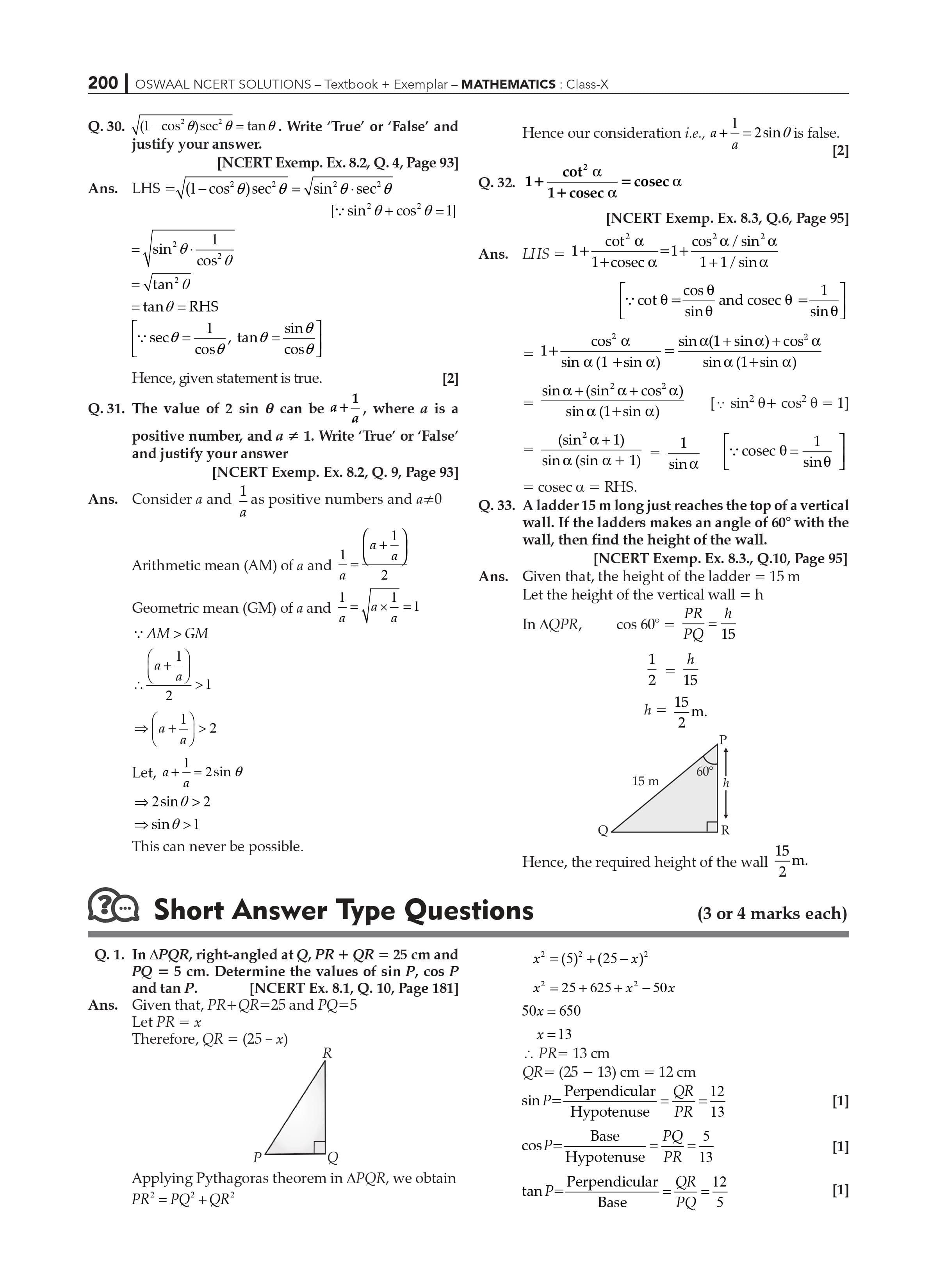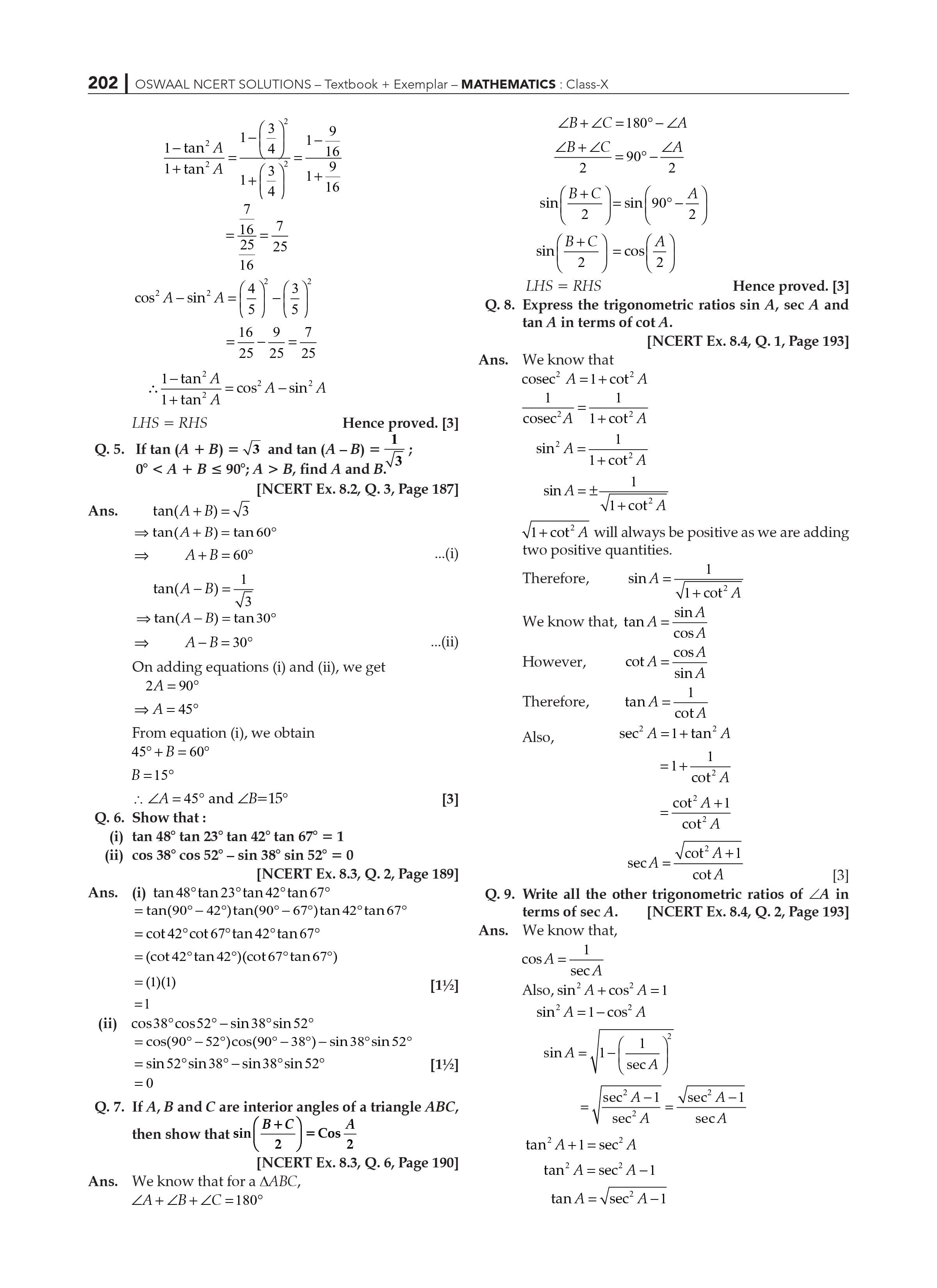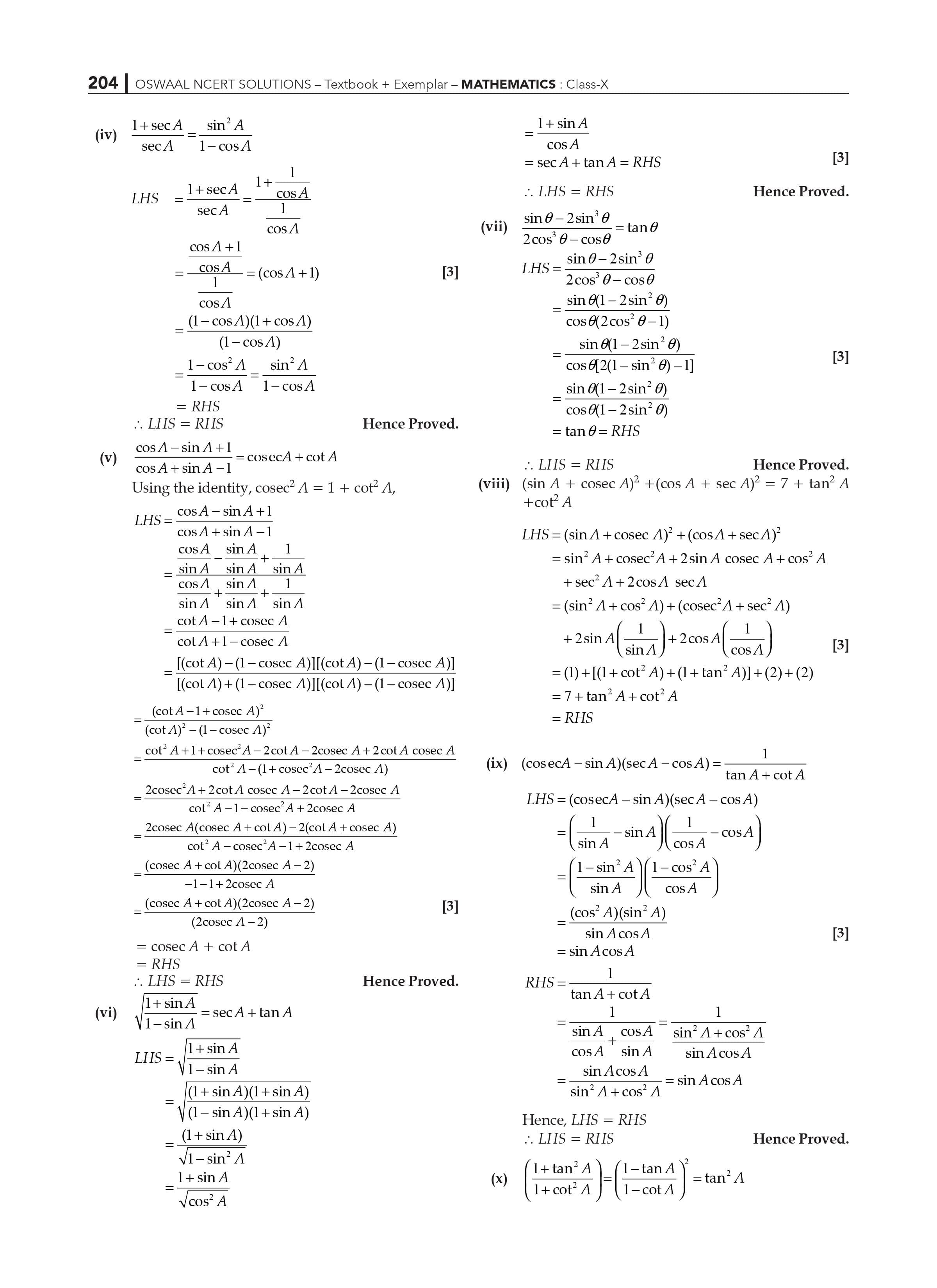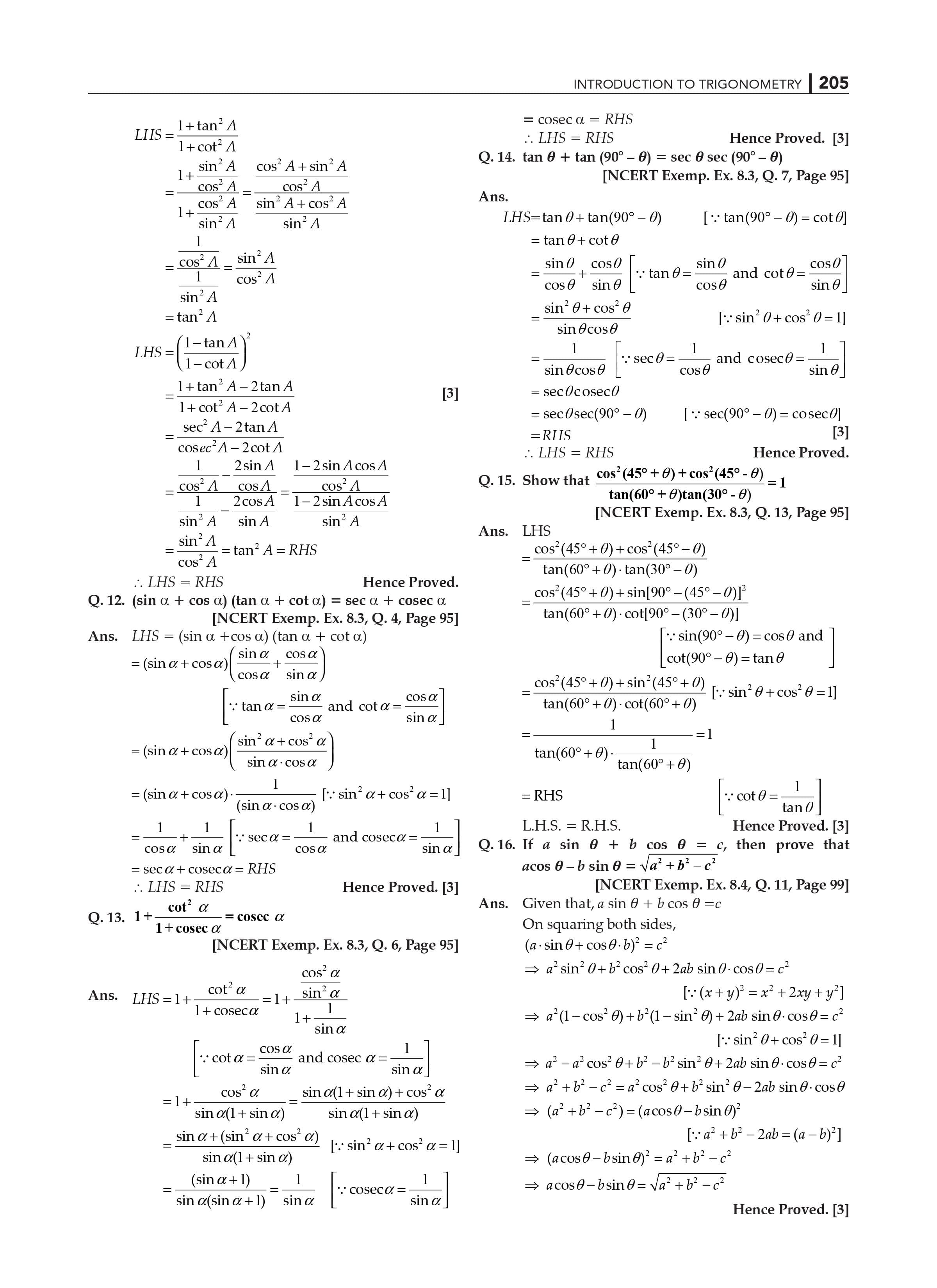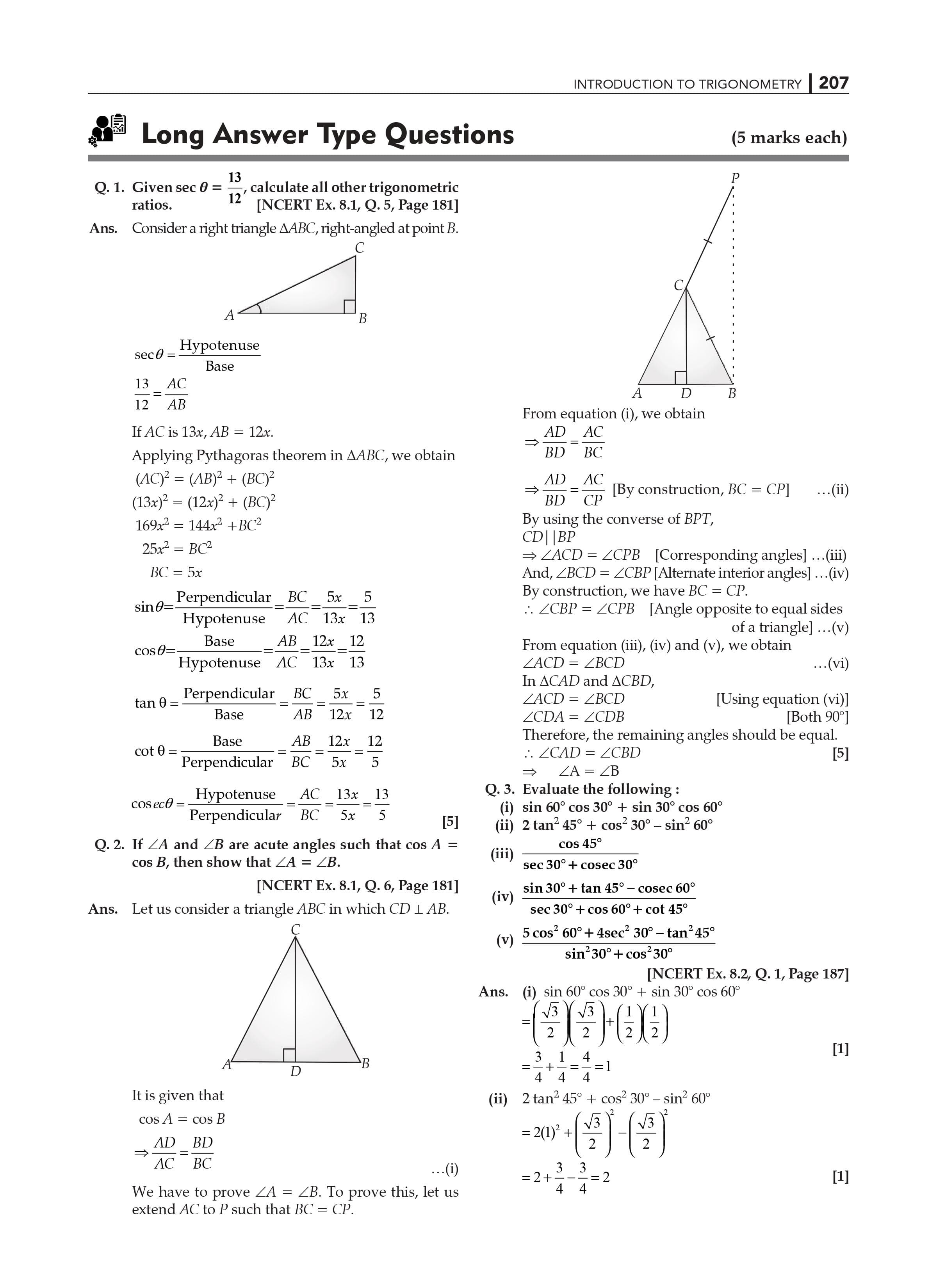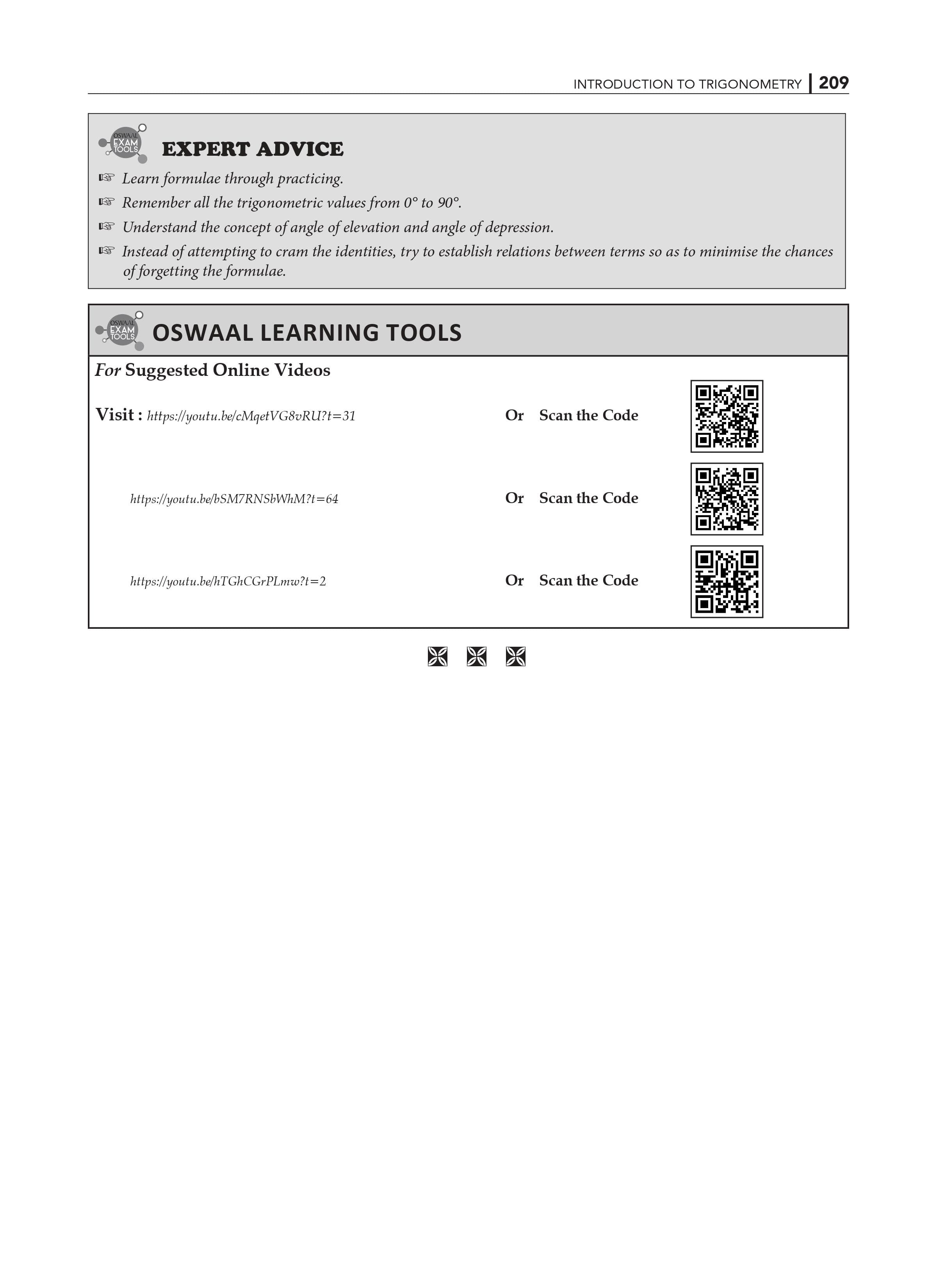The Beginning: In previous classes, you've already learned about triangles, specifically right triangles. In this chapter, we'll look at some trigonometric ratios of the angle, ratios of the sides of a right triangle to their acute angles. Also defined are the trigonometric ratios for angles of measure 0o and 90o.

We'll calculate trigonometric ratios for some specific angles and establish trigonometric identities, which are identities involving these ratios.

Identities Trigonometric: In previous classes, you learned about the concept of ratio. Certain ratios involving the sides of a right triangle are now referred to as trigonometric ratios. Using various Trigonometry functions, the topic is explained with appropriate examples.

Some Specific Angles' Trigonometric Ratios: You're already familiar with constructing angles of 30o, 45o, 60o, and 90o from Geometry. The values of the trigonometric ratios for these angles and 0o will be found in this section. It uses appropriate examples to explain 45o, 30o, and 60o trigonometric ratios and 0o and 90o trigonometric ratios.

Complementary Angle Trigonometric Ratios: When the sum of two angles equals 90 degrees, they are said to be complementary. Various formulas for solving numerical problems involving trigonometric ratios are discussed in this topic.

Identities Trigonometric: You may recall that when an equation is true for all values of the variables involved, it is called an identity. Similarly, if an equation involving trigonometric ratios of an angle is true for all values of the angles involved, it is called a trigonometric identity. We will prove one trigonometric identity in this section and then use it to prove other useful trigonometric identities.

Conclusion: The conclusion is a condensed version of the concepts that includes all of the key points that you'll need to remember to solve numerical problems related to the chapter.

As a result, these NCERT Solutions for Class 10 Maths Chapter 8 will assist students in comprehending various types of questions and their responses, as well as key shortcuts and diagrammatic representations. The PDFs of the NCERT Solutions for Class 10 Maths Chapter 8 are written in simple English. If students fully comprehend these solutions, they will be able to solve complex problems with ease. The faculty has organized the NCERT Solutions for Class 10 clearly to help students improve their problem-solving skills. Students can use the study materials available in our Class 10 books to understand Introduction to Trigonometry better.

 Extra Information: NCERT Exemplar for Class 10 Maths Chapter 8 CBSE Notes for Class 10 Maths Chapter 8

Que1. In the first term exam of Class 10 Maths, list the frequently asked topics from Chapter 8 of NCERT Solutions.

Answer1. In the first term exam of Class 10 Maths, topics such as introduction to trigonometry, trigonometric identities, trigonometric ratios of some specific angles, trigonometric ratios of complementary angles, and trigonometric identities are frequently asked.

Que2. Why should we use OSWAAL to get NCERT Solutions for Class 10 Maths Chapter 8?

Answer2. For the questions in NCERT Solutions for Class 10 Maths Chapter 8, Oswaal CBSE Books Class 10 provides the most accurate answers. Experts explain the solutions to this chapter's problems in detail, with neat diagrams where necessary.

Que3. Is it important to study NCERT Solutions for Class 10 Maths Chapter 8 for the exam 2023?

Answer3. Yes, all of the chapters in NCERT Solutions for Class 10 Maths are crucial for 2023 exams and higher grades. Students should practice all of the questions in NCERT Solutions for Class 10 Maths Chapter 8 to get good grades.